# Lets play a game Lets play a game

• Slides: 18Let’s play a game…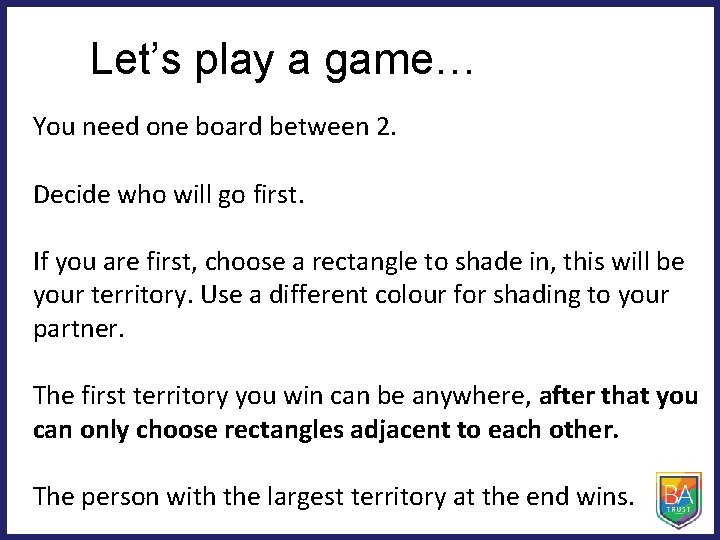Let’s play a game… You need one board between 2. Decide who will go first. If you are first, choose a rectangle to shade in, this will be your territory. Use a different colour for shading to your partner. The first territory you win can be anywhere, after that you can only choose rectangles adjacent to each other. The person with the largest territory at the end wins.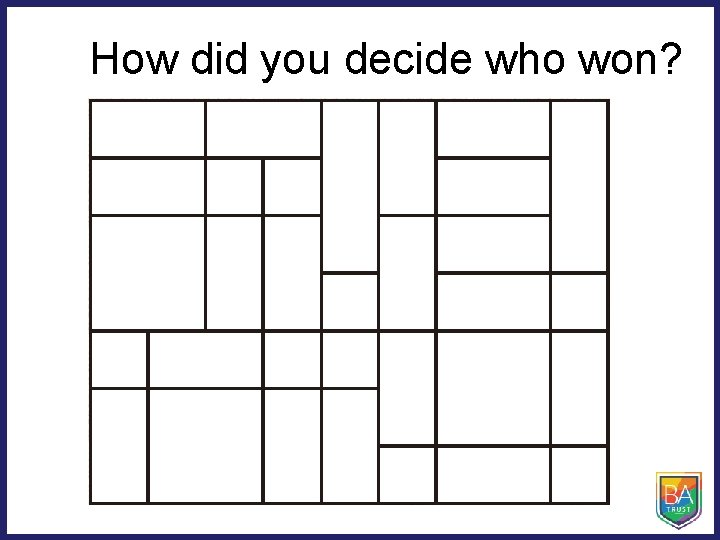How did you decide who won?How can we compare the amount of space? This is a game after 4 people have played How can we tell who has won?How can you compare the sizes of the territories? Spend a couple of minutes thinking quietly on your own about how you would compare the sizes. Think about how you would explain your reasoning. Now turn to your partner and compare your strategies.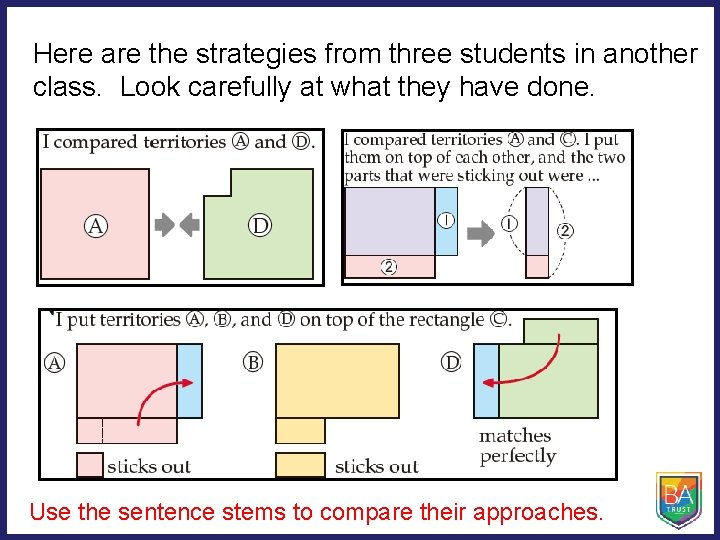Here are the strategies from three students in another class. Look carefully at what they have done. Use the sentence stems to compare their approaches.Did any of you use a different approach to compare the sizes? Can you explain clearly?As shown below, we can subdivide the territories into squares with 1 cm sides. Which territory has the most space, and by how much?You will recall from previous years, that the amount of space is called the area. The area is the number of 1 cm squares that can be placed inside the shape. The area of a square with 1 cm sides is 1 square centimetre, written as 1 cm 2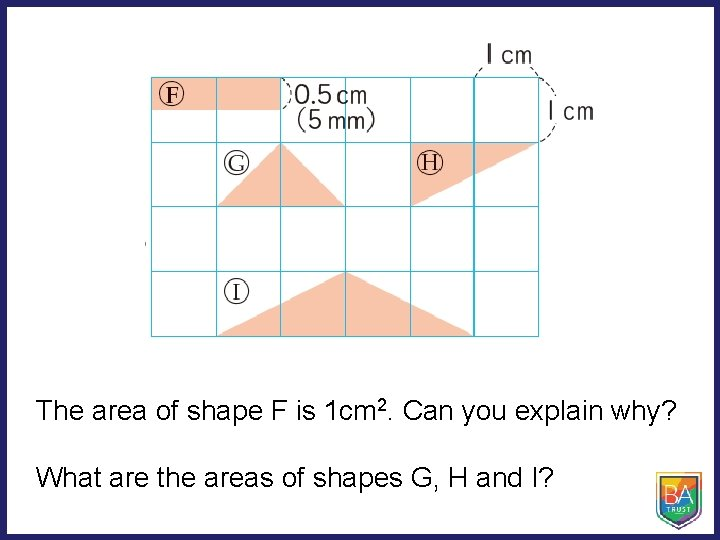The area of shape F is 1 cm 2. Can you explain why? What are the areas of shapes G, H and I?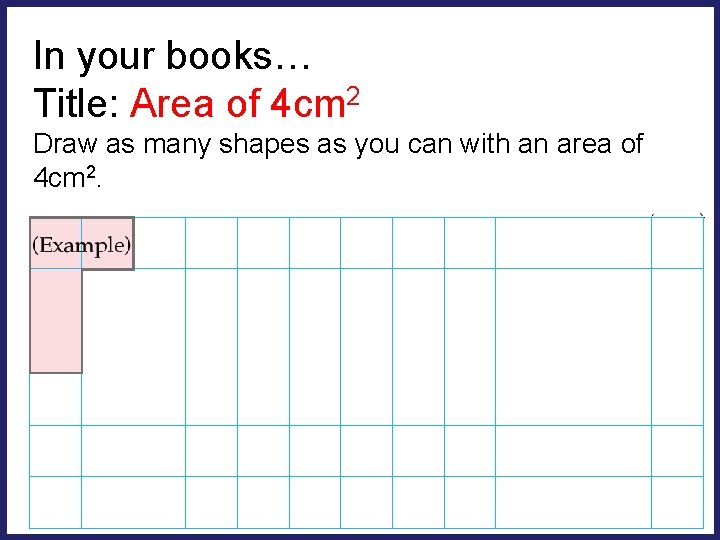In your books… Title: Area of 4 cm 2 Draw as many shapes as you can with an area of 4 cm 2.How many different ways can you find the areas of the rectangles and square below?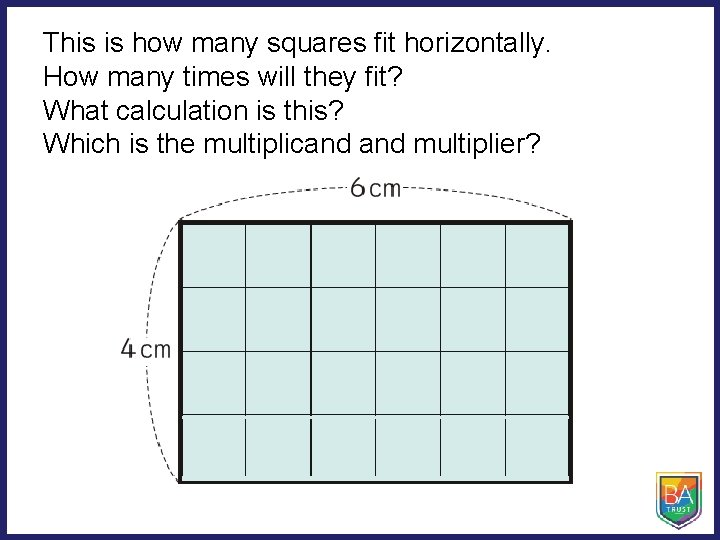This is how many squares fit horizontally. How many times will they fit? What calculation is this? Which is the multiplicand multiplier?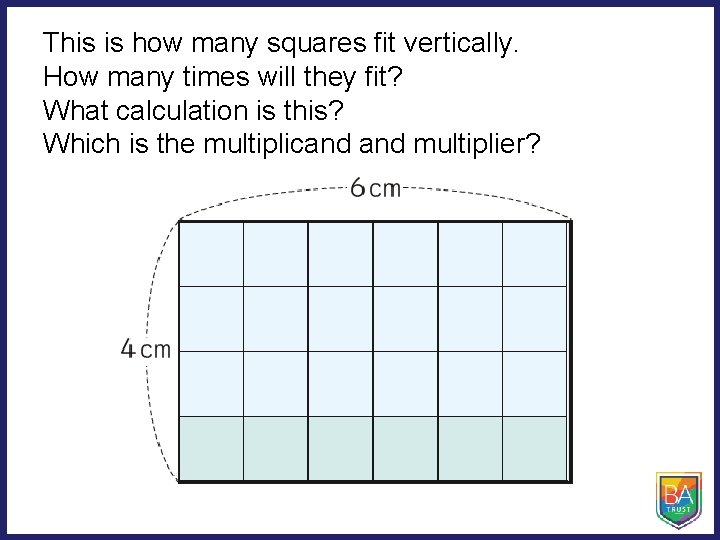This is how many squares fit vertically. How many times will they fit? What calculation is this? Which is the multiplicand multiplier?On your whiteboards Find the areas of these rectangles: 3 cm 6 cm 1. 5 cm 2 cm 4 cm 8 cm 12 cm 1 cm Can you write the dimensions of some other rectangles that have an area of 12 cm 2?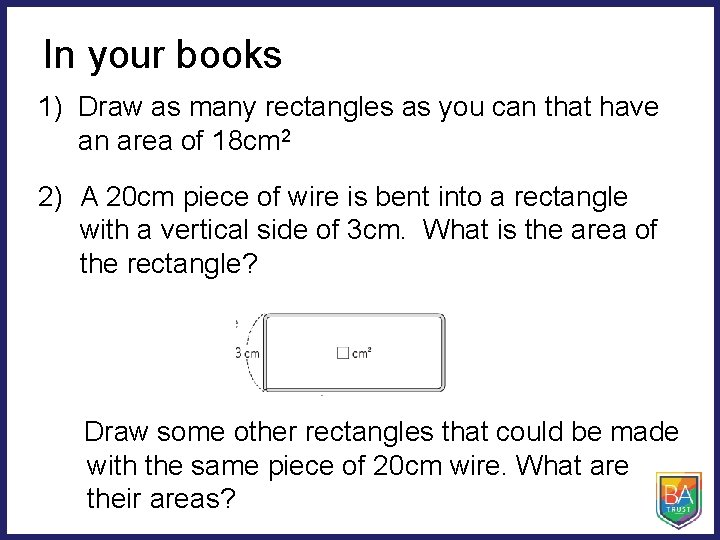In your books 1) Draw as many rectangles as you can that have an area of 18 cm 2 2) A 20 cm piece of wire is bent into a rectangle with a vertical side of 3 cm. What is the area of the rectangle? Draw some other rectangles that could be made with the same piece of 20 cm wire. What are their areas?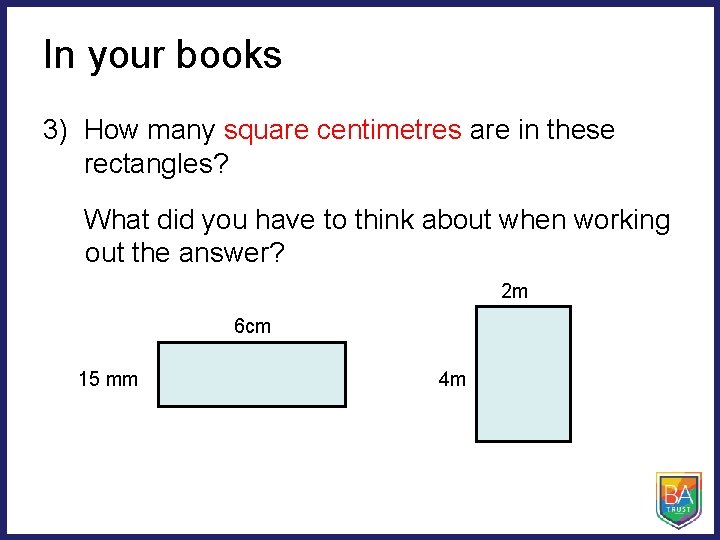In your books 3) How many square centimetres are in these rectangles? What did you have to think about when working out the answer? 2 m 6 cm 15 mm 4 m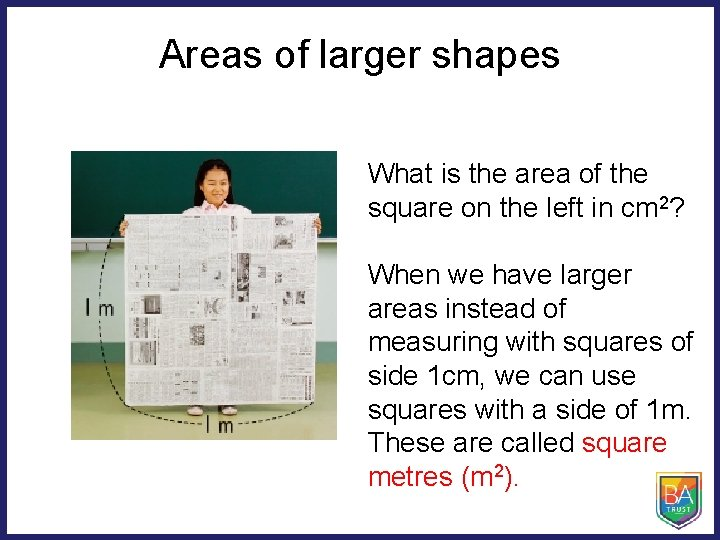Areas of larger shapes What is the area of the square on the left in cm 2? When we have larger areas instead of measuring with squares of side 1 cm, we can use squares with a side of 1 m. These are called square metres (m 2).